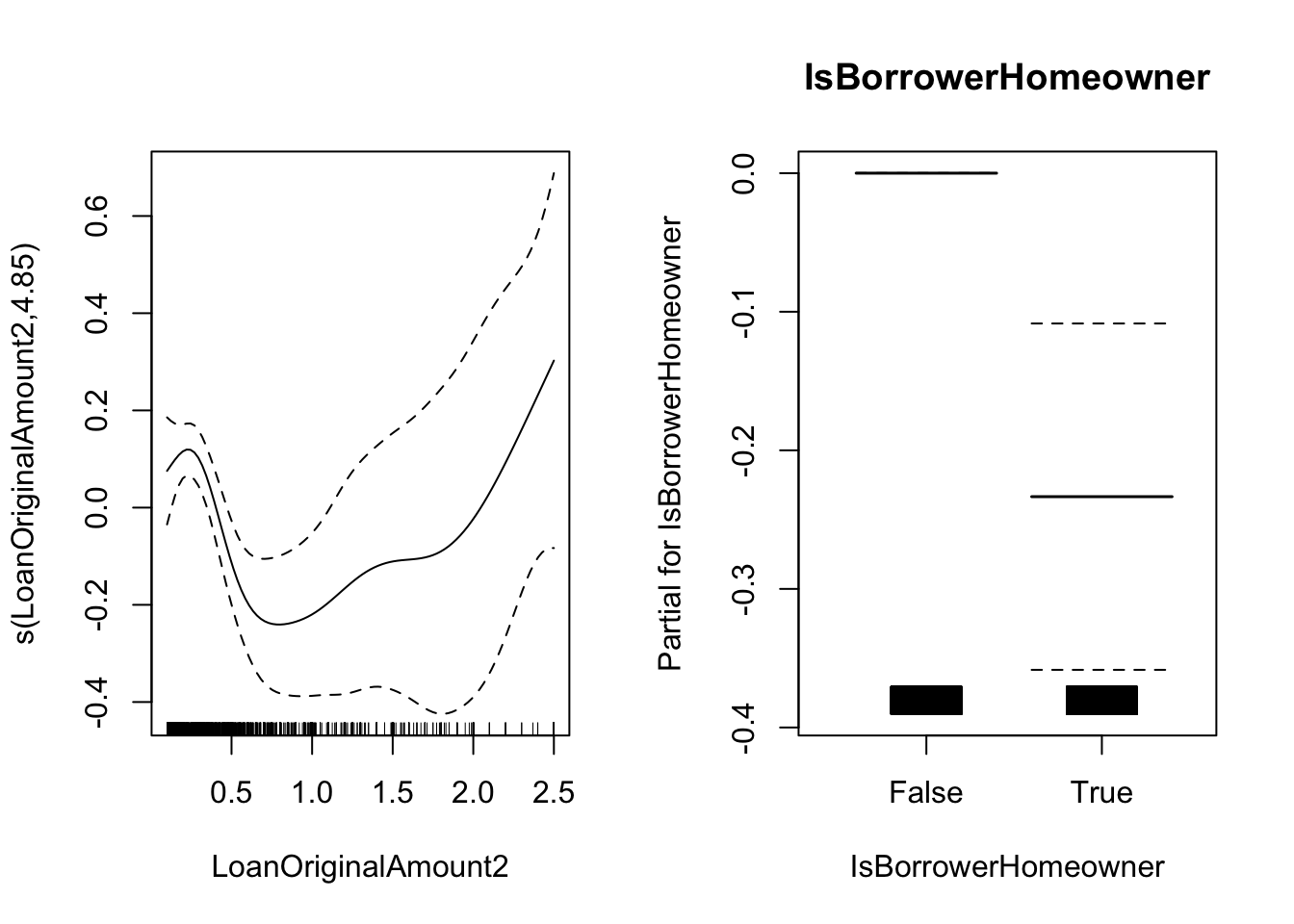## 3.9 Bonus track 1: Additive Cox model

The Cox PH model assumes a linear effect of the predictors. If the true effect is highly nonlinear this can lead to a nonproportinal hazards or misleading statistical conclusions.

One alternative approach is to use an Additive Cox model (Hastie and Tibshirani 1990) of the form

$h(t, \textbf X) = h_0(t) e^{\sum_{j=1}^p f_j(\textbf X_j)}$ with $$f_j$$ being an unknown and smooth function.

In order to estimate this model one could use the mgcv package as follows

m4 <- mgcv::gam(time ~ s(LoanOriginalAmount2) + IsBorrowerHomeowner,
data = loan_filtered, family = "cox.ph", weights = status)
summary(m4)
##
## Family: Cox PH
##
## Formula:
## time ~ s(LoanOriginalAmount2) + IsBorrowerHomeowner
##
## Parametric coefficients:
##                         Estimate Std. Error z value Pr(>|z|)
## IsBorrowerHomeownerTrue -0.23344    0.06248  -3.736 0.000187 ***
## ---
## Signif. codes:  0 '***' 0.001 '**' 0.01 '*' 0.05 '.' 0.1 ' ' 1
##
## Approximate significance of smooth terms:
##                          edf Ref.df Chi.sq  p-value
## s(LoanOriginalAmount2) 4.853  5.857  26.91 0.000206 ***
## ---
## Signif. codes:  0 '***' 0.001 '**' 0.01 '*' 0.05 '.' 0.1 ' ' 1
##
## Deviance explained = 0.818%
## -REML =  10848  Scale est. = 1         n = 4923
plot(m4, pages = 1, all.terms = TRUE)Note the change in the sintaxis compared with the previous examples. The status indicator in used in the weights argument.

### References

Hastie, T., and R. Tibshirani. 1990. Generalized Additive Models. London: Chapman; Hall.